# Wavelet Denoising¶

This numerical tour uses wavelets to perform non-linear image denoising. $\newcommand{\dotp}{\langle #1, #2 \rangle}$ $\newcommand{\qandq}{\quad\text{and}\quad}$ $\newcommand{\qwhereq}{\quad\text{where}\quad}$ $\newcommand{\qifq}{ \quad \text{if} \quad }$ $\newcommand{\ZZ}{\mathbb{Z}}$ $\newcommand{\RR}{\mathbb{R}}$ $\newcommand{\pa}{\left(#1\right)}$ $\newcommand{\si}{\sigma}$ $\newcommand{\Nn}{\mathcal{N}}$ $\newcommand{\Bb}{\mathcal{B}}$ $\newcommand{\EE}{\mathbb{E}}$ $\newcommand{\norm}{\|#1\|}$ $\newcommand{\abs}{\left|#1\right|}$ $\newcommand{\choice}{ \left\{ \begin{array}{l} #1 \end{array} \right. }$ $\newcommand{\al}{\alpha}$ $\newcommand{\la}{\lambda}$ $\newcommand{\ga}{\gamma}$ $\newcommand{\Ga}{\Gamma}$ $\newcommand{\La}{\Lambda}$ $\newcommand{\si}{\sigma}$ $\newcommand{\Si}{\Sigma}$ $\newcommand{\be}{\beta}$ $\newcommand{\de}{\delta}$ $\newcommand{\De}{\Delta}$ $\newcommand{\phi}{\varphi}$ $\newcommand{\th}{\theta}$ $\newcommand{\om}{\omega}$ $\newcommand{\Om}{\Omega}$

In :
using PyPlot
using NtToolBox
# arequire("NtToolBox")


## Image Denoising¶

We consider a simple generative model of noisy images $F = f_0+W$ where $f_0 \in \RR^N$ is a deterministic image of $N$ pixels, and $W$ is a Gaussian white noise distributed according to $\Nn(0,\si^2 \text{Id}_N)$, where $\si^2$ is the variance of noise.

The goal of denoising is to define an estimator $\tilde F$ of $f_0$ that depends only on $F$, i.e. $\tilde F = \phi(F)$ where $\phi : \RR^N \rightarrow \RR^N$ is a potentially non-linear mapping.

Note that while $f_0$ is a deterministic image, both $F$ and $\tilde F$ are random variables (hence the capital letters).

The goal of denoising is to reduce as much as possible the denoising error given some prior knowledge on the (unknown) image $f_0$. A mathematical way to measure this error is to bound the quadratic risk $\EE_w(\norm{\tilde F - f_0}^2)$, where the expectation is computed with respect to the distribution of the noise $W$.

Image loading and adding Gaussian Noise For real life applications, one does not have access to the underlying image $f_0$. In this tour, we however assume that $f_0$ is known, and $f = f_0 + w\in \RR^N$ is generated using a single realization of the noise $w$ that is drawn from $W$. We define the estimated deterministic image as $\tilde f = \phi(f)$ which is a realization of the random vector $\tilde F$.

First we load an image $f_0 \in \RR^N$ where $N=n \times n$ is the number of pixels, and then display it.

In :
n = 256
name = "NtToolBox/src/data/flowers.png"
imageplot(f0, "Original image")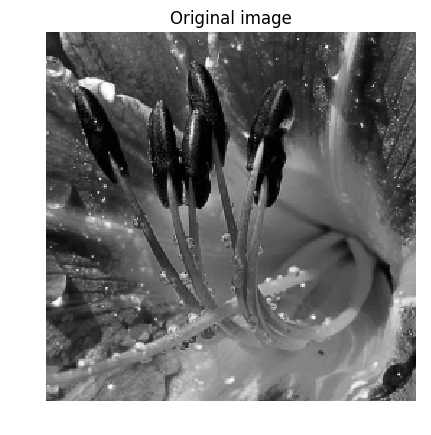libpng warning: iCCP: known incorrect sRGB profile
libpng warning: iCCP: known incorrect sRGB profile
libpng warning: iCCP: known incorrect sRGB profile

Out:
PyObject <matplotlib.text.Text object at 0x329420a10>

Standard deviation $\si$ of the noise.

In :
sigma = .08;


Then we add Gaussian noise $w$ to obtain $f=f_0+w$.

In :
using Distributions
f = f0 + sigma.*rand(Normal(), size(f0), size(f0));


Display the noisy image.

In :
imageplot(clamP(f), string("Noisy, SNR = ", string(snr(f0,f)) ))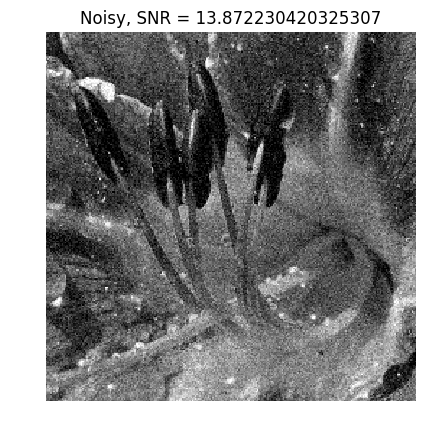Out:
PyObject <matplotlib.text.Text object at 0x329f71210>

## Hard Thresholding in Wavelet Bases¶

A simple but efficient non-linear denoising estimator is obtained by thresholding the coefficients of $f$ in a well chosen orthogonal basis $\Bb = \{\psi_m\}_m$ of $\RR^N$.

In the following, we will focuss on a wavelet basis, which is efficient to denoise piecewise regular images.

The hard thresholding operator with threshold $T \geq 0$ applied to some image $f$ is defined as $$S_T^0(f) = \sum_{\abs{\dotp{f}{\psi_m}}>T} \dotp{f}{\psi_m} \psi_m = \sum_m s_T^0(\dotp{f}{\psi_m}) \psi_m$$ where the hard thresholding operator is $$s_T^0(\alpha) = \choice{ \alpha \qifq \abs{\al}>T, \\ 0 \quad\text{otherwise}. }$$

The denoising estimator is then defined as $$\tilde f = S_T^0(f).$$

Display the function $s_T^0(\al)$ for $T=1$.

In :
thresh_hard = (u, t) -> u.*(abs(u) .> t)
alpha = linspace(-3, 3, 1000)
plot(alpha, thresh_hard(alpha, 1))
axis("equal")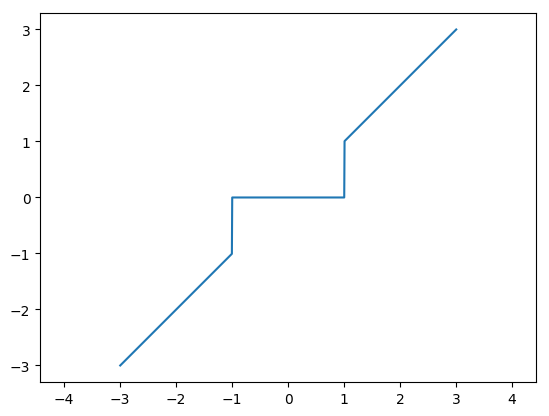Out:
(-3.3,3.3,-3.3,3.3)

Parameters for the orthogonal wavelet transform.

In :
h = [0, .482962913145, .836516303738, .224143868042, -.129409522551]
h = h./norm(h)
Jmin = 2;


First we compute the wavelet coefficients $a$
of the noisy image $f$.

In :
a = perform_wavortho_transf(f, Jmin, +1, h);


Display the noisy coefficients.

In :
plot_wavelet(a, Jmin);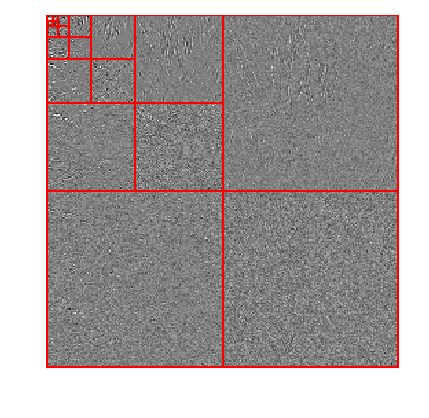Select the threshold value, that should be proportional to the noise level $\si$.

In :
T = 3*sigma;


Hard threshold the coefficients below the noise level to obtain $a_T(m)=s_T^0(a_m)$.

In :
aT = thresh_hard(a, T);


Display the thresholded coefficients.

In :
plot_wavelet(aT, Jmin);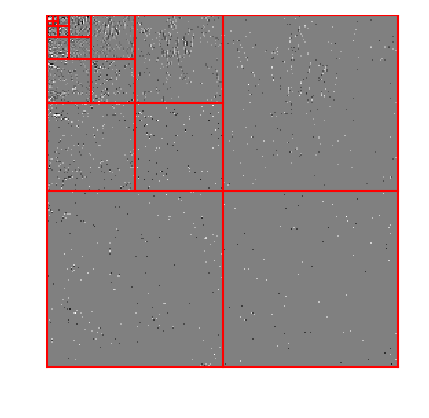Reconstruct the image $\tilde f$ from these noisy coefficients.

In :
fHard = perform_wavortho_transf(aT, Jmin, -1, h);


Display the denoising result.

In :
imageplot(clamP(fHard), string("Hard, SNR = ", string(snr(f0, fHard))) )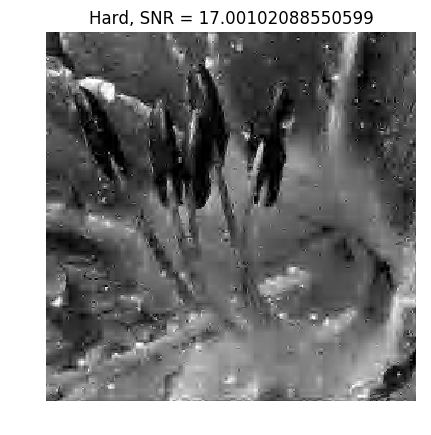Out:
PyObject <matplotlib.text.Text object at 0x32a9900d0>

## Wavelet Denoising with Soft Thesholding¶

The estimated image $\tilde f$ using hard thresholding. suffers from many artifacts. It is possible to improve the result by using soft thresholding, defined as $$\tilde f = S_T^1(f) = \sum_m s_T^1(\dotp{f}{\psi_m}) \psi_m$$ $$\qwhereq s_T^1(\alpha) = \max\pa{0, 1 - \frac{T}{\abs{\alpha}}}\alpha.$$

Display the soft thresholding function $s_T^1(\al)$.

In :
thresh_soft = (u, t) -> max(1 - t./abs(u), 0).*u
alpha = linspace(-3, 3, 1000)
plot(alpha, thresh_soft(alpha, 1))
axis("equal");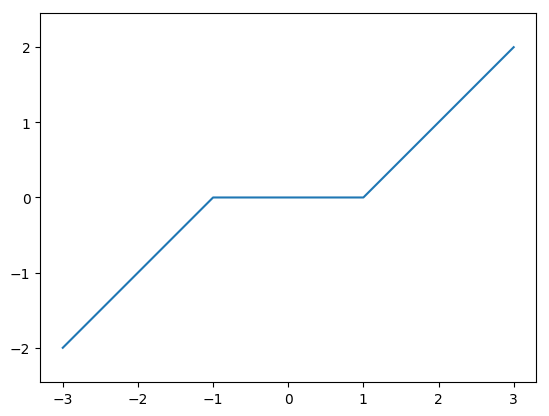Select the threshold.

In :
T = 3/2*sigma;


Perform the soft thresholding.

In :
aT = thresh_soft(a, T);


To slightly improve the soft thresholding performance, we do not threshold the coefficients corresponding to coarse scale wavelets.

In :
aT[1:2^Jmin, 1:2^Jmin] = a[1:2^Jmin, 1:2^Jmin];


Re-construct the soft thresholding estimator $\tilde f$.

In :
fSoft = perform_wavortho_transf(aT, Jmin, -1, h);


Display the soft thresholding denoising result.

In :
imageplot(clamP(fSoft), string("Soft, SNR=", string(snr(f0, fSoft))) )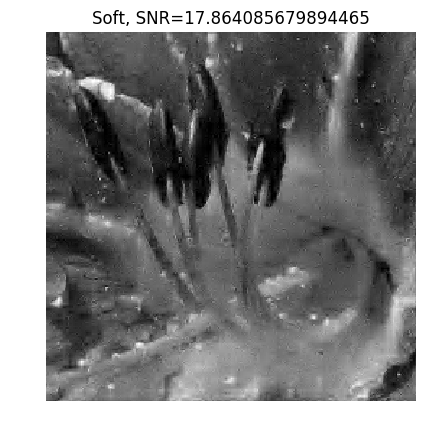Out:
PyObject <matplotlib.text.Text object at 0x329515a90>

One can prove that if the non-linear approximation error $\norm{f_0-S_T(f_0)}^2$ decays fast toward zero when $T$ decreases, then the quadratic risk $\EE_w( \norm{f-S_T(f)}^2 )$ also decays fast to zero when $\si$ decays. For this result to hold, it is required to select the threshold value according to the universal threshold rule $$T = \si \sqrt{2\log(N)}.$$

Determine the best threshold $T$ for both hard and soft thresholding. Test several (T) values in $[.8\sigma, 4.5\sigma[$, and display the empirical SNR $-10\log_{10}(\norm{f_0-\tilde f}/\norm{f_0})$ What can you conclude from these results ? Test with another image.

In :
include("NtSolutions/denoisingwav_2_wavelet_2d/exo1.jl")

could not open file /Users/gpeyre/Dropbox/github/numerical-tours/julia/Exos\denoisingwav_2_wavelet_2d\exo1.jl

in include_from_node1(::String) at /Applications/Julia-0.5.app/Contents/Resources/julia/lib/julia/sys.dylib:?

## Translation Invariant Denoising with Cycle Spinning¶

Orthogonal wavelet transforms are not translation invariant. It means that the processing of an image and of a translated version of the image give different results.

Any denoiser can be turned into a translation invariant denoiser by performing a cycle spinning. The denoiser is applied to several shifted copies of the image, then the resulting denoised image are shifted back to the original position, and the results are averaged.

This corresponds to defining the estimator as $$\tilde f = \frac{1}{M} \sum_{i=1}^{M} T_{-\de_i} \circ S_T(f) \circ T_{\de_i}$$ where $S_T$ is either the hard or soft thresholding, and $T_\de(f)(x) = f(x-\de)$ is the translation operator, using periodic boundary conditions. Here $(\de_i)_i$ is a set of $M$ discrete translation. Perfect invariance is obtained by using all possible $N$ translatation, but usually a small number $M \ll N$ of translation is used to obtain approximate invariance.

Number $m$ of translations along each direction so that $M = m^2$.

In :
m = 4;


Generate a set of shifts $(\de_i)_i$.

In :
include("NtToolBox/src/ndgrid.jl")
(dY, dX) = meshgrid(0:m-1, 0:m-1)
delta = cat(2, reshape(dX, m*m, 1), reshape(dY, m*m, 1));

could not open file /Users/gpeyre/Dropbox/github/numerical-tours/julia/ndgrid.jl

in include_from_node1(::String) at /Applications/Julia-0.5.app/Contents/Resources/julia/lib/julia/sys.dylib:?

To avoid storing all the translates in memory, one can perform a progressive averaging of the translates by defining $f^{(0)}=0$ and then $$\forall\, i=1,\ldots,M, \quad f^{(i)} = \pa{1-\frac{i}{n}} f^{(i-1)} + \frac{i}{n} T_{-\de_i} \circ S_T(f) \circ T_{\de_i}$$ One then has $\tilde f = f^{(M)}$ after $M$ steps.

Initialize the denoised image $f^{(0)}=0$.

In :
fTI = zeros(n, n)
T = 3*sigma
index = 1
for i in 1:m*m
fS = circshift(f, delta[i, :])
a = perform_wavortho_transf(fS, Jmin, 1, h)
aT = thresh_hard(a, T)
fS = perform_wavortho_transf(aT, Jmin, -1, h)
fS = circshift(fS, -delta[i, :])
fTI = i/(i + 1.0).*fTI + 1.0/(i + 1).*fS
index += 1
end

UndefVarError: delta not defined

in macro expansion; at ./In:5 [inlined]
in anonymous at ./<missing>:?
In :
imageplot(clamP(fTI), string("TI, SNR = ", string(snr(f0,fTI))) )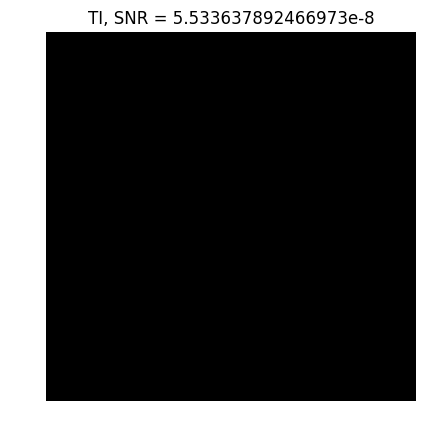Out:
PyObject <matplotlib.text.Text object at 0x32a12e1d0>

# Exercises¶

Exercise 1: Study the influence of the number $m$ of shift on the denoising quality.

Exercise 2: Select the best threshold $T$ for the translation invariant denoising.

Exercise 3: Implement a block-thresholding method, that threshold coefficient in square blocks according to the energy of each of these blocks.